﻿ Unique Fixed Points on OWC for Self-Maps with Generalized Contractive Type Conditions in CMSPublications are Open
Access in this journal
Article Versions
Export Article
• Normal Style
• MLA Style
• APA Style
• Chicago Style
Research Article
Open Access Peer-reviewed

### Unique Fixed Points on OWC for Self-Maps with Generalized Contractive Type Conditions in CMS

K. Prudhvi
American Journal of Applied Mathematics and Statistics. 2023, 11(2), 61-62. DOI: 10.12691/ajams-11-2-3
Received March 05, 2023; Revised April 12, 2023; Accepted April 24, 2023

### Abstract

In this paper, we obtain fixed point theorem for OWC (Occasionally Weakly Compatible) self-mappings satisfying a generalized contractive type condition in CMS (Cone Metric Space). Our results are generalizing and improving some of the well known comparable results existing in this literature.

### 1. Introduction

The fixed point theory is an important area of non-linear analysis. Recently Huang and Zhang 1 generalized the concept of metric space into cone metric space. And the concept of cone metric space initially introduced by the Huang and Zhang 1 and they replacing the real numbers by an ordered Banach space and they also proved some fixed point theorems in cone metric space. Later on many authors inspired with this results extended these results in many way (see for e.g., [2-11]) in different types of contractive type conditions in cone metric space. Recently Bhatt and Chandra 6. In this paper, we proved a unique common fixed point theorem for OWC satisfying a generalized contractive condition in CMS.

### 2. Preliminaries

The following important preliminaries are useful in our main results.

Definition 2.1. Let L be a real Banach space. A subset M of L is called a cone iff

(i) M is a non-empty and closed also M ≠ {0},

(ii) u, v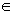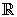, and α, β ≥ 0, u, vM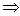uα + vβM,

(iii) M ∩ (-M) = {0}.

Given a cone M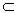L, we define a partial ordering ≤ with respect to M by α ≤ β iff β – αM. A cone M is called normal if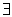a number K> 0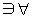α, βM, 0 ≤ α ≤ β implies ||α||≤ K ||β||.

The least positive number satisfying the above inequality is called the normal constant of M, while α << β stands for β – αinterior of M.

Definition 2.2. Let X be a nonempty set. And suppose that the mapping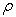: X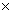X→ L satisfying the following conditions:

(1). 0 ≤(α, β) for all α, β∈X and(α,β) = 0 if and only if α = β,

(2).(α, β) =(β, α) for all α, β∈X;

(3).(α, β)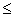(α, γ) +(γ, β) for all α, β, γ∈X.

Thenis called a cone metric on X and (X,) is called a CMS.

Definition 2.3. Let (X,) be CMS. We say that {xn} is

(i) a convergent sequence if for any b>>0, there exists a natural number N such that for all n>N,(xn, x) <<b, for some fixed x in X. We denote this xn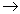x (as n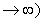.

(ii) a Cauchy sequence if for every b in M with b>>0, there exists a natural number N such that for all n, m>N,(xn,xm)<<b.

Definition 2.4. A cone metric space (X,) is said to be complete if every Cauchy sequence is convergent.

Definition 2.5 9. Let A and B be self-mappings of a set X. If q = Aα = Bα for some α in X, then α is called a coincidence point of A and B, and q is called a point of coincidence of A and B.

Proposition 2.1. Let A and B be OWC self-mappings of a set X if and only if there is a point α in X which is coincidence point of A and B at which A and B are commute.

Lemma 2.1. Let X be a set, A and B are OWC self-mappings of X. If A and B have a unique point of coincidence q = Aα = Bα, then q is the unique.

### 3. Main Results

In this section, we prove a unique common fixed point theorem for OWC self-mappings in CMS (Cone Metric Space). Ours result is improvement and generalization of the results of 6.

Let Ф: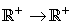be a function satisfying the condition Ф(t) < t for each t > 0.

Now we prove the our main theorem

Theorem 3.1. Let (X,) be a CMS and M be a normal cone. And suppose that l, m are two self- mappings of X and they satisfy the following conditions:

(i)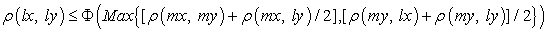for each x, yX.

(ii) l and m are OWC.

Then l and m have a unique common fixed point.

Proof. Given (by (ii)) l and m are occasionally weakly compatible, then there exists point pX such that lp = mp, lmp = mlp. We claim that, lp is the unique common fixed point of l and m. First we ascertain that lp is a fixed point of p. For if, llp ≠ lp, then by (i) we get that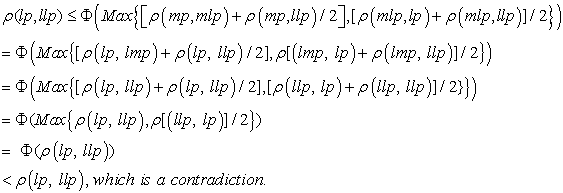Therefore, llp = lp and llp = mlp = lp. Thus lp is a common fixed point of l and m.

Uniqueness: suppose that p, qX such that lp = mp = p and lq = mq = q and p ≠ q. Then by (i) we get that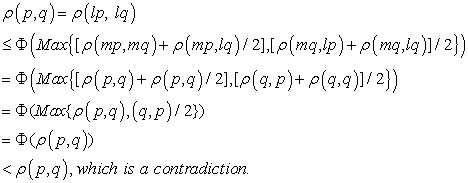Therefore, p = q. Therefore, l and m have a unique common fixed point. This completes the proof of the theorem.

### 4. Conclusion

Our results are more general then the results of 6.

### Conflict of Interest

Author has declared there is no conflict of interest.

### Acknowledgements

Author grateful to the reviewers to review this article and great suggestions for improvement.

### References

  L.G. Huang, X. Zhang, Cone metric spaces and fixed point theorems of contractive mappings J. Math. Anal. Appl. 332(2) (2007), 1468-1476. In article View Article  M. Abbas and G. Jungck, Common fixed point results for non commuting mappings without continuity in cone metric spaces, J. Math. Anal. Appl. 341(2008) 416-420. In article View Article  M. Abbas, B. E. Rhoades, Fixed and periodic point results in cone metric spaces, Appl. Math. Lett. 21(2008): 511-515. In article View Article  I. Altun, B. Durmaz, Some fixed point theorems on ordered cone metric spaces, Rend. Circ. Mat. Palermo 58(2009), 319-325. In article View Article  M.A.Al-Thagafi and Naseer Shahzad, Generalized I-non expansive self maps and invariant approximations, Acta Mathematica Sinica, 24(2008), 867-876 In article View Article  Arvind Bhatt and Harish Chandra, Occasionally weakly compatible mappings in cone metric space, Applied Mathematical Sciences, Vol. 6, no. 55, (2012), 2711-2717. In article  Guangxing Song, Xiaoyan Sun, Yian Zhao, Guotao Wang, New common fixed point theorems for maps on cone metric spaces, Appl. Math. Lett. 32(2010) 1033-1037. In article View Article  G.Jungck and B.E. Rhoades, Fixed point theorems for occasionally weakly compatible mappings, Fixed Point Theory, 7(2006), 286-296. In article  G.Jungck and B.E. Rhoades, Fixed point theorems for occasionally weakly compatible mappings, Erratum, Fixed Point Theory, 9(2008), 383-384. In article  Ö. Özer, A. Shatarah, A kind of fixed point theorem on the complete C*- Algebra valued s-metric spaces, Asia Mathematika, vol.4., Iisue .1, (2020), 53-62. In article  K. Prudhvi, A Unique Common Fixed Point Theorem for a Metric Space with the Property (E.A), American Journal of Applied Mathematics and Statistics, Vol.11., No.1, (2023), 11-12. In article View ArticleThis work is licensed under a Creative Commons Attribution 4.0 International License. To view a copy of this license, visit http://creativecommons.org/licenses/by/4.0/